﻿ 基于心电脉搏的无创连续血压检测系统的设计

# 基于心电脉搏的无创连续血压检测系统的设计Design of Noninvasive Continuous Blood Pressure Detection System Based on ECG and Pulse

Abstract: In this paper, based on the continuous monitoring of blood pressure, a set of non-invasive contin-uous blood pressure detection system based on ECG and pulse was designed and implemented. Adopting the monitoring mode of hardware terminal + mobile APP, hardware terminal realizes ECG and pulse signal acquisition and processing; mobile APP realizes the recognition of characteristic points of ECG and pulse signal, establishes blood pressure model, then calculates pulse transit time, and finally calculates blood pressure value. The system effectively achieves non-invasive continuous blood pressure measurement, and provides an effective solution for the treatment and prevention of hypertension diseases.

1. 引言

2. 系统测量原理

1878年，Moens与Korteweg经过实验提出了脉搏波速度(pulse wavevalociy, PWV)  的公式：

$PWV=K\sqrt{\frac{Eh}{\rho D}}$ (1)

$E={E}_{0}\mathrm{exp}\left(\alpha p\right)$ (2)

$PWV=K\sqrt{\frac{{E}_{0}h}{\rho D}\mathrm{exp}\left(\alpha p\right)}$ (3)

$PWV=\frac{L}{PTT}$ (4)

$p=\frac{1}{\alpha }\left(\mathrm{ln}\frac{{L}^{2}\rho d}{h{E}_{0}}-2\mathrm{ln}PPT\right)$ (5)

$\mathrm{ln}PTT={\sum }_{n=1}^{\infty }{\left(-1\right)}^{n-1}×\frac{{\left(PPT-1\right)}^{n}}{n}$ (6)

$P=a×PWTT+b$ (7)

3. 系统总体方案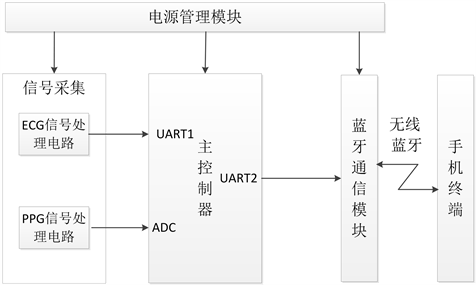Figure 1. System block diagram

4. 系统硬件设计

4.1. 心电采集模块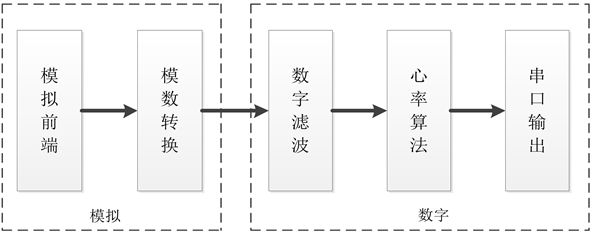Figure 2. BMD101 internal processing flow chart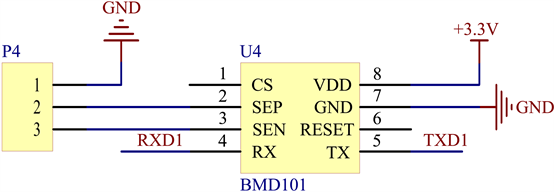Figure 3. BMD101 ECG detection circuit schematic

4.2. 脉搏采集模块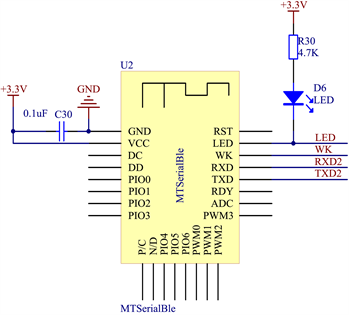Figure 4. Bluetooth module circuit schematic

5. 系统软件设计

5.1. 终端软件设计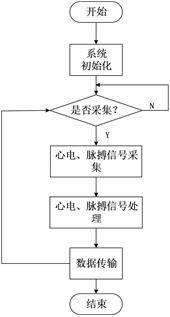Figure 5. Terminal signal acquisition

5.2. 手机APP设计

ECG与PPG信号的特征点获取实现对ECG信号R波点与PPG信号主波点定位。其处理步骤如下：

1) 选定一段信号为数组φ(n)，φ(n)对相邻数据做差值，即进行一阶导得dx(n)：

$dx\left(n\right)=\phi \left(n\right)-\phi \left(n-1\right)$ (8)

2) 根据 $dx\left(n\right)\ast dx\left(n-1\right)$ 的值来判断峰点与谷点，如果其值小于0，则记录该点；

3) 对所有的峰点与谷点进行识别，从峰点开始检测，如果第一个点是谷点，则跳过。寻找所有满足比左邻近最小谷点值(初始值为信号最小值，指从上次检测到的有效峰值点开始，到当前峰点之间的最小谷值点)、比右邻近谷点都高于一定阈值的有效峰点，并且在一个有效峰点检测过程中，检测到的峰点为两次有效检测所有可能的峰点中的最大值点。阈值一般取信号最大值与最小值之差的三分之一，可自适应调整；

4) 对检测到的有效峰点再次进行判断，判断两个有效峰值点之间的距离是否在一定的区间，若满足条件的的则即为目标峰值点，而不符合要求的情况下，舍去其中较小的那个有效峰值点。其中区间一般可选取采样频率的1/2到1倍之间，可自适应调整。

6. 系统测试Table 1. System measurement and cuff sphygmomanometer measurement results statistics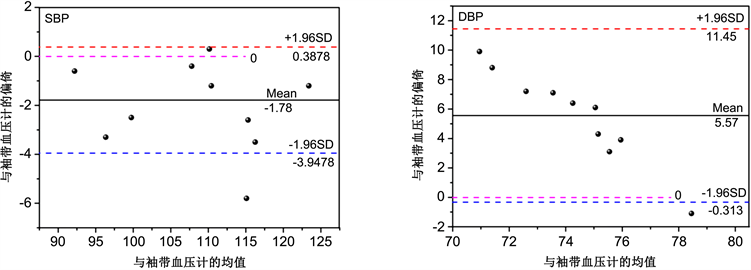Figure 6. Bland-Altman analysis of system blood pressure and cuff blood pressure

7. 总结

  杨秀环, 梁华杰, 梁秀生, 等. 连续无创血压监测技术的研究进展[J]. 现代诊断与治疗, 2016, 27(18): 3364-3366.

 陈巨兰. 高血压病的临床研究与治疗进展[J]. 中国医学文摘(内科学), 2000(6): 753-755.

 高伟. 基于脉搏波的连续血压监测仪器的设计[D]: [硕士学位论文]. 天津: 天津大学, 2016.

 尹赛男. 基于脉搏波传导时间的无创连续血压测量研究[D]: [硕士学位论文]. 沈阳: 东北大学, 2012.

 Chen, W., Kobayashi, T., Ichikawa, S., et al. (2000) Continuous Estimation of Systolic Blood Pressure Using the Pulse Arrival Time and Inter-mittent Calibration. Medical & Biological Engineering & Computing, 38, 569-574.
https://doi.org/10.1007/BF02345755

 李章俊. 基于光电容积脉搏波描记法的无创连续血压测量[J]. 中国生物医学工程学报, 2012, 31(4): 607-614.

Top